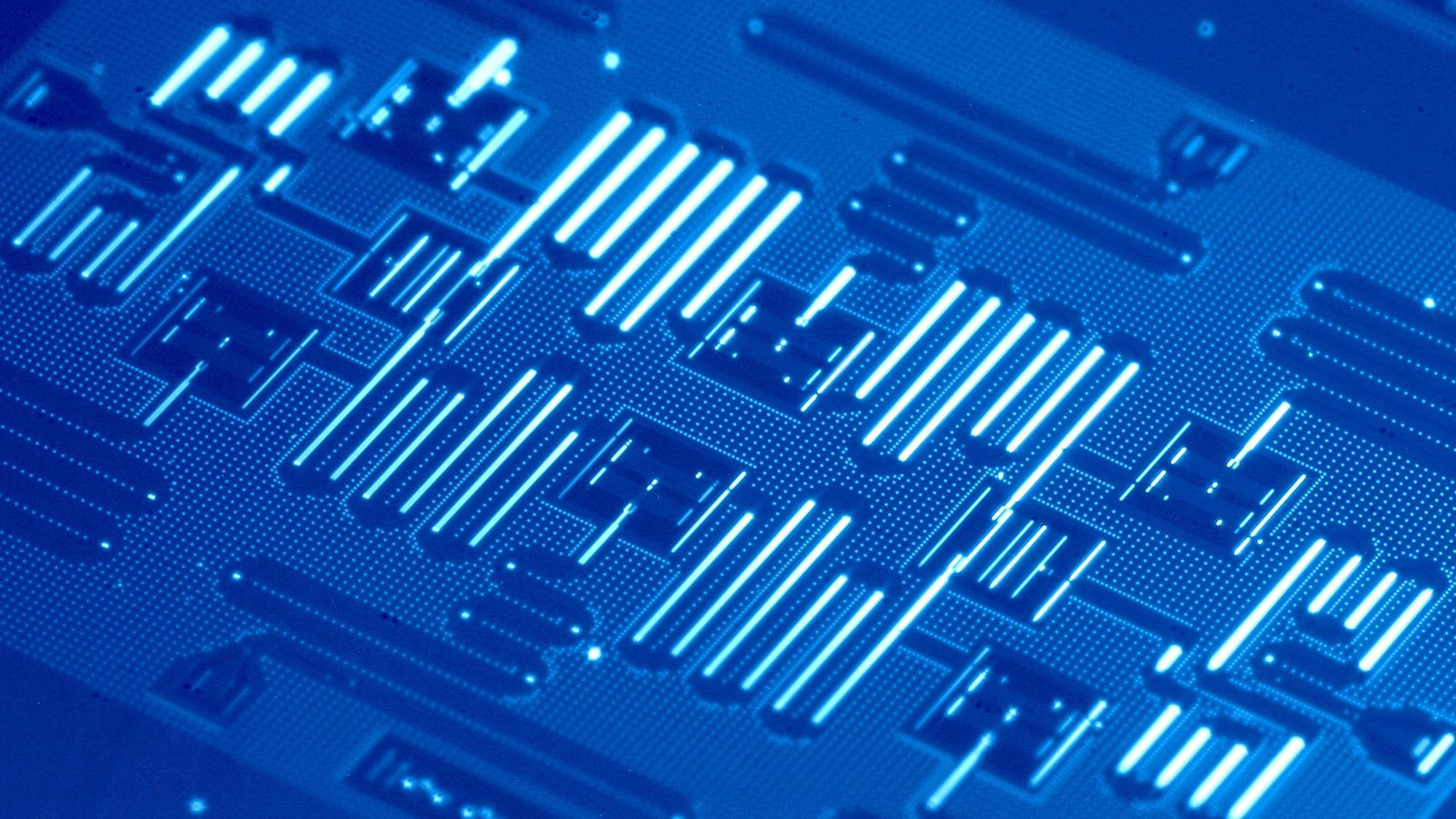Technical

# How to manipulate a superconducting qubit?###### How to manipulate a superconducting qubit?

Of many quantum computing frameworks, the one that is claimed to be much advanced than most of its contemporaries is the one that uses superconducting qubits because of a very simple reason: superconducting quantum hardware implements quantum computing in such a way that quantum effects become macroscopic in nature. However, these artificially synthesized atoms acting as qubits have to be operated in an extremely low noise environment. When microwave pulse of calculated frequency and amplitude is shot on the set-up of the qubit, its state changes corresponding to the pulse parameters. And this is how we manipulate the qubit.

### Idealization : Qubits have flavor?

The typology of qubits is rather the variance of physical support ranging from photon, electron, neutron to Josephson Junction. Physical implementation of Josephson Junction to construct superconducting qubits is where we are particularly interested in. Ideally, these qubits have three different archetypes based on how they leverage three different properties : 1) Charge, 2) Current, 3) Energy, whereas in reality some hybrids do also exist (Example: Transmon qubit). They are called superconducting-charge, superconducting-flux and superconducting-phase qubits respectively. The characterization of different frameworks results in equally different qubit-manipulation methods.

For superconducting-charge qubit, we map uncharged superconducting island (Q=0) to be the logical zero-state and charged superconducting island (Q=2e, one extra cooper pair in theory, much more in practice) to be the logical one-state (learn more about superconductors). Transmon qubit is a derivative of charge qubit, further configured for experimental benefit.

### Big Picture: Qubits in the cloud.

Many of us by now have been introduced to IBM Quantum and have run one or two jobs in real quantum devices. These quantum computers at IBM are heartily dependent on Transmon qubits. When you attempt to run a job, it is queued via cloud for being executed in a device therein IBM quantum. You might have often seen a hanging device with gold-plated wires and loops being pointed as a quantum computer. This golden chandelier is in-fact a superconducting dilution fridge that holds the quantum circuitry at temperature of around 15 milli-Kelvin. At the inverted apex you can find some shields in which superconducting qubit-chip with read-out resonators rest.

The qubits you will find there are not natural quantum objects but are constructed with intricate circuitry to act as a logical qubit. An understanding of electromagnetic oscillator makes it easier to grasp how these qubits are manufactured.

### Anharmonic Oscillators as Superconducting Qubits.

A classical oscillator is harmonic in nature and is composed of inductor and capacitor. A capacitor stores energy in an electric field; an inductor stores energy in a magnetic field. Now when electrons move with respect to voltage in a circuit with capacitor and inductor, they form cooper-pair (2 electrons) in very low temperature and have in principle, zero resistance. The total spin of these cooper pairs are integer numbers and this allow them to act as bosons. In contrary to fermions used as qubits, they can occupy a single quantum energy level in an effect known as Bose-Einstein condensate. A discrete number of magnetic flux quanta penetrates a superconducting loop, similarly to the discrete atomic energy levels in the Bohr model: a square of the wave function amplitude at some point in space is the probability of finding a charge carrier there, hence the square of the amplitude corresponds to the classical charge distribution. Voila! We have our macroscopic quantum system. Well, not yet. Remember how the classical oscillator was harmonic? Unfortunately, harmonic oscillator is not a good candidate for qubit. We need to isolate two energy levels from all identical levels to use it as a quBIT(0 and 1). To do that we introduce a non-linear tunable inductor which will change its inductance based on the amount of stored energy. Josephson Junction is an example of such inductor that turns the artificial atom into an anharmonic artificial atom.

Why anharmonic? Because we need to make sure that the electron is not leaking to other energy levels beyond the two we referred as state one and state zero. Anharmonicity increases the gap between relevant two levels and further levels of excitation. So this is how a physical layout of the artificial qubit is set.

### How do we manipulate superconducting qubits?

Now that we understand what happens beyond algebra, bras and kets, how about we design a gate for our qubit? Qiskit Pulse is a language to code for the gate. Gates are basically microwave pulses applied within tiny time frames to excite the qubit. Superconducting qubits allow arbitrary rotations in the Bloch sphere with pulsed microwave signals, thus implementing arbitrary single qubit gates. The qubits are arranged in a drive and we calculate the drive frequency and amplitude to tune our pulse. First, the qubit frequency is found by sweeping a range of frequencies and looking for signs of absorption gives a rough estimate of the qubit frequency. Then using the frequency we shoot multiple pulse rays with a range of expected amplitude. When fitted into a curve function, we will be able to find the approximate amplitude for intended state of the qubit. Scheduling a pulse experiment as a job to be run on the measured drive results in the expected state. The Qiskit Textbook provides a detailed guide on how to calibrate a single qubit gate.

Application of superconducting qubit is an active area of research. It has a small decoherence time and for being artificially synthesized no two qubits can have identical dependencies. Even if this framework proves not to be the best in the future, this is the one that helped Google to reach the first milestone of quantum supremacy.